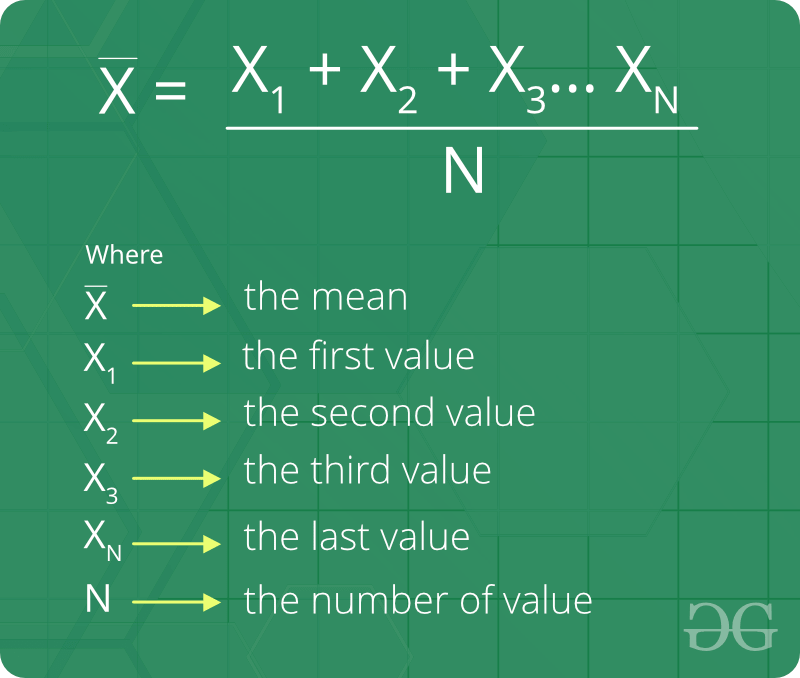# Arithmetic Mean

• Last Updated : 02 Jul, 2022

Arithmetic mean, also called the average or average value, is the quantity obtained by summing two or more numbers or variables and then dividing by the number of numbers or variables. The arithmetic mean is important in statistics.
For example, Let’s say there are only two quantities involved, the arithmetic mean is obtained simply by adding the quantities and dividing by 2.

Some interesting fact about Arithmetic Mean :1. The mean of n numbers x1, x2, . . ., xn is x. If each observation is increased by p, the mean of the new observations is (x + p).
2. The mean of n numbers x1, x2, . . ., xn is x. If each observation is decreased by p, the mean of the new observations is (x – p).
3. The mean of n numbers x1, x2, . . ., xn is x. If each observation is multiplied by a nonzero number p, the mean of the new observations is px.
4. The mean of n numbers x1, x2, . . ., xn is x. If each observation is divided by a nonzero number p, the mean of the new observations is (x/p).

Formula of Arithmetic Mean:How to find Arithmetic Mean ?
Given three integers A, B and N the task is to find N Arithmetic means between A and B. We basically need to insert N terms in an Arithmetic progression. where A and B are first and last terms.

Examples:

```Input : A = 20 B = 32 N = 5
Output : 22 24 26 28 30
The Arithmetic progression series is
20 22 24 26 28 30 32

Input : A = 5  B = 35  N = 5
Output : 10 15 20 25 30```

Approach :
Let A1, A2, A3, A4……An be N Arithmetic Means between two given numbers A and B . Then A, A1, A2 ….. An, B will be in Arithmetic Progression. Now B = (N+2)th term of the Arithmetic progression. So :

Finding the (N+2)th term of the Arithmetic progression Series, where d is the Common Difference

```B = A + (N + 2 - 1)d
B - A  = (N + 1)d```

So the Common Difference d is given by.

`d = (B - A) / (N + 1)`

We have the value of A and the value of the common difference(d), now we can find all the N Arithmetic Means between A and B.

## C++

 `// C++ program to find n arithmetic``// means between A and B``#include ``using` `namespace` `std;` `// Prints N arithmetic means between``// A and B.``void` `printAMeans(``int` `A, ``int` `B, ``int` `N)``{``    ``// calculate common difference(d)``    ``float` `d = (``float``)(B - A) / (N + 1);` `    ``// for finding N the arithmetic``    ``// mean between A and B``    ``for` `(``int` `i = 1; i <= N; i++)``        ``cout << (A + i * d) << ``" "``;``}` `// Driver code to test above``int` `main()``{``    ``int` `A = 20, B = 32, N = 5;``    ``printAMeans(A, B, N);``    ``return` `0;``}`

## Java

 `// java program to illustrate``// n arithmetic mean between``// A and B``import` `java.io.*;``import` `java.lang.*;``import` `java.util.*;` `public` `class` `GFG {` `    ``// insert function for calculating the means``    ``static` `void` `printAMeans(``int` `A, ``int` `B, ``int` `N)``    ``{``        ``// Finding the value of d Common difference``        ``float` `d = (``float``)(B - A) / (N + ``1``);` `        ``// for finding N the Arithmetic``        ``// mean between A and B``        ``for` `(``int` `i = ``1``; i <= N; i++)``            ``System.out.print((A + i * d) + ``" "``);``    ``}` `    ``// Driver code``    ``public` `static` `void` `main(String args[])``    ``{``        ``int` `A = ``20``, B = ``32``, N = ``5``;``        ``printAMeans(A, B, N);``    ``}``}`

## Python3

 `# Python3 program to find n arithmetic``# means between A and B` `# Prints N arithmetic means``# between A and B.``def` `printAMeans(A, B, N):` `    ``# Calculate common difference(d)``    ``d ``=` `(B ``-` `A) ``/` `(N ``+` `1``)``    ` `    ``# For finding N the arithmetic``    ``# mean between A and B``    ``for` `i ``in` `range``(``1``, N ``+` `1``):``        ``print``(``int``(A ``+` `i ``*` `d), end ``=` `" "``)` `# Driver code``A ``=` `20``; B ``=` `32``; N ``=` `5``printAMeans(A, B, N)` `# This code is contributed by Smitha Dinesh Semwal`

## C#

 `// C# program to illustrate``// n arithmetic mean between``// A and B``using` `System;` `public` `class` `GFG {` `    ``// insert function for calculating the means``    ``static` `void` `printAMeans(``int` `A, ``int` `B, ``int` `N)``    ``{``        ``// Finding the value of d Common difference``        ``float` `d = (``float``)(B - A) / (N + 1);` `        ``// for finding N the Arithmetic``        ``// mean between A and B``        ``for` `(``int` `i = 1; i <= N; i++)``            ``Console.Write((A + i * d) + ``" "``);``    ``}` `    ``// Driver code``    ``public` `static` `void` `Main()``    ``{``        ``int` `A = 20, B = 32, N = 5;``        ``printAMeans(A, B, N);``    ``}``}``// Contributed by vt_m`

## PHP

 ``

## Javascript

 ``

Output:

`22 24 26 28 30`

Time Complexity: O(N)

Auxiliary Space: O(1)

Basic Program related to Arithmetic Mean

My Personal Notes arrow_drop_up